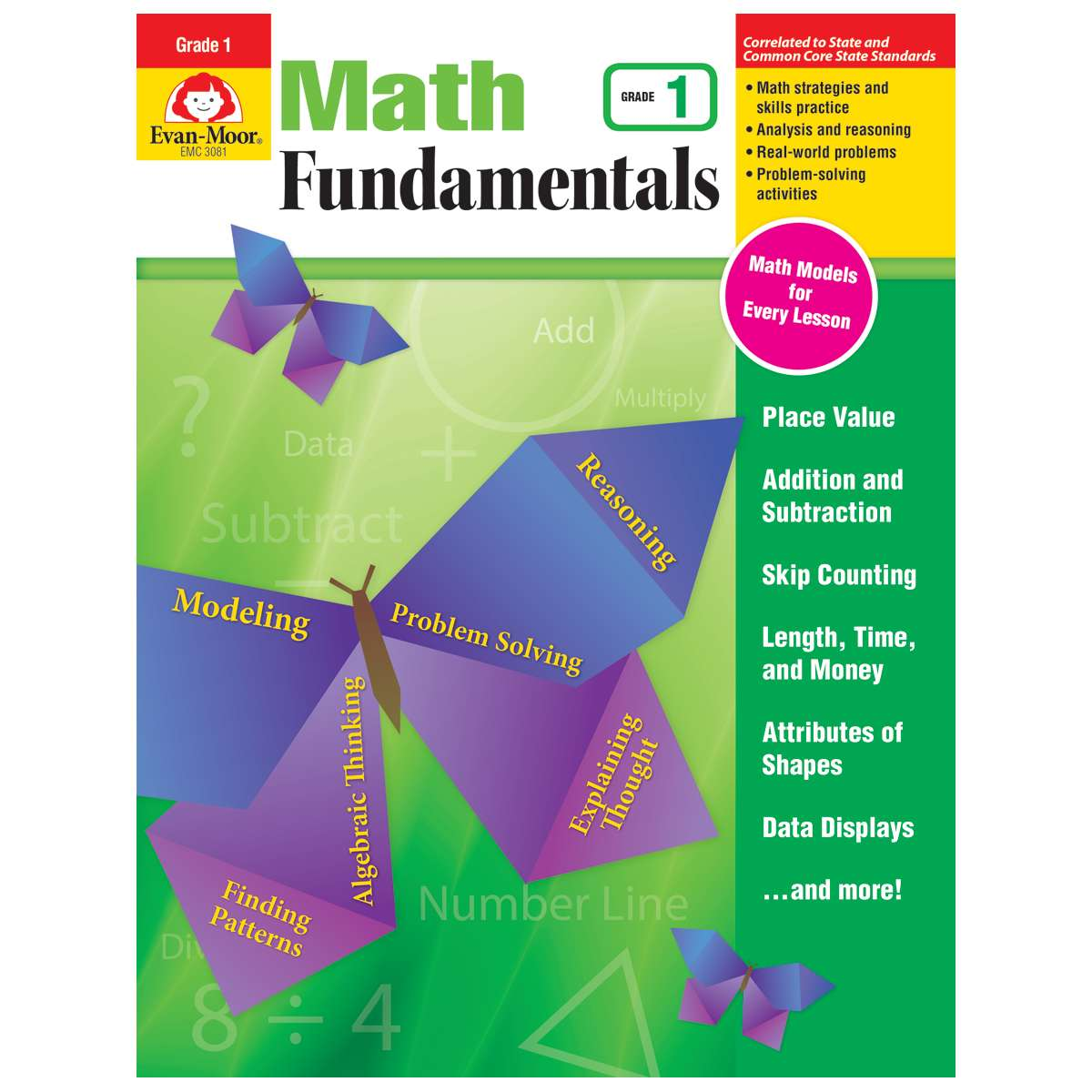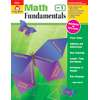•### Product Media

Product Number: EMC3081
Comprehensive but not complicated! Math Fundamentals helps your first grade students navigate the new math. Math Models and “...
Quantity

Comprehensive but not complicated! Math Fundamentals helps your first grade students navigate the new math. Math Models and “think” questions, plenty of skill practice, and real-world problems guide students in thinking through, analyzing, and solving problems.

To help you target instruction, each unit clearly lists the standards information, mathematical practices, and skills covered.

Within a unit, math lessons are presented simply. Every math lesson includes:
• A Math Models reference page that shows students strategies for solving problems. This is helpful for parents and students transitioning to new math.
• Skill practice pages that progress in difficulty.
• A culminating problem-solving activity that leads students through the process of solving a real-life problem.
• Word problems
• Operations and number relationships
• Add and subtract within 20
• Equivalency and unknown numbers
• Counting and number sequences
• Place value: two-digit numbers
• Place value: addition and subtraction
• Measuring length
• Time
• Charts and graphs
• Money
• Geometry
Designed to support new math programs, Math Fundamentals is the perfect easy-to-use supplement to your Common Core math or current math curriculum.

Better together! Use Math Fundamentals with Daily Math Practice for complementary skill practice that ensures your students master grade-level math.

This resource contains teacher support pages, reproducible student pages, and an answer key.
EMC3081